# Limits Continuity Differentiability And Differentiation Set-4

Go back to  'SOLVED EXAMPLES'

Example - 11

Let  f\left( x \right) = \left\{ \begin{align}&x\sin \frac{1}{x} & \,\,,\,\,\,\,x \ne 0\\&\,\,\,\,\,\,\,\,0\, & \,\,,\,\,\,\,x = 0\end{align} \right\}\,{\rm{ and\; }}g(x) = \left\{ \begin{array}{l}{x^2}\sin \frac{1}{x}\qquad\quad, & x \ne 0\\\,\,\,\,\,\,\,\,\,\,0 \qquad\qquad , & x = 0\end{array} \right\}.

Evaluate the continuity and differentiability of  $$f(x)$$ and $$g(x)$$ .

Solution:   We will first try to graphically understand the behaviour of these two functions and      then verify our results analytically.  Notice that no matter what the argument of the sin function is, its magnitude will always remain between –1 and 1.

Therefore,

\begin{align}&{\rm{}}\left| {\,x\sin \frac{1}{x}\,} \right| \le \,\,|x|\\\\&and{\rm{ }}\left| {\,{x^2}\sin \frac{1}{x}\,} \right| \le \,\,|x{|^2}\end{align}

This means that the graph of $$x\sin \frac{1}{x}$$  will always lie between the lines $$y = \pm{x}$$ and  the graph $${x^2}\sin \frac{1}{x}$$ will always lie between the two curves $$y = \pm {x^2}$$

Also, notice that as | x | increases, $$\frac{1}{x}$$  decreases in a progressively slower manner while when   | x | is close to 0, the increase in $$\frac{1}{x}$$   is very fast (as | x | decreases visualize the graph of $$y = \frac{1}{x}$$ ).

This means that near the origin, the variation in the graph of sin $$\frac{1}{x}$$  will be extremely rapid because the successive zeroes of the graph will become closer and closer. As we keep on increasing x, the variation will become slower and slower and the graph will ‘spread out’. For example, for x > $$\frac{1}{\pi }$$ there will be no finite root of the function. Only when $$x \to \infty$$  will  $$\sin \frac{1}{x}$$ again approach 0.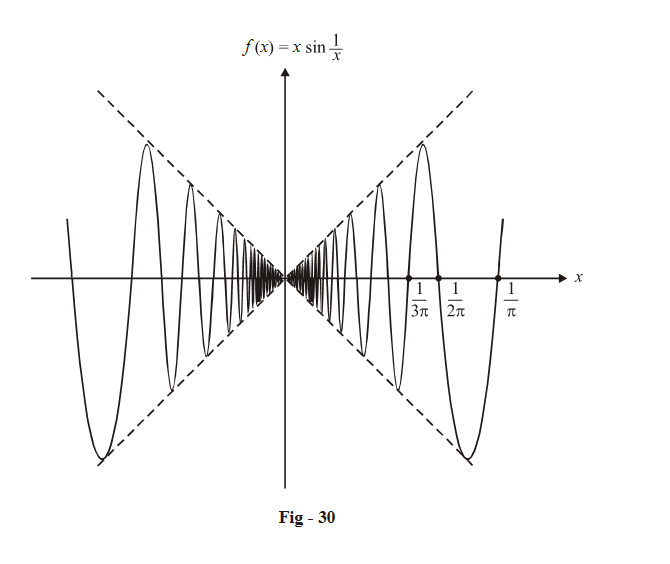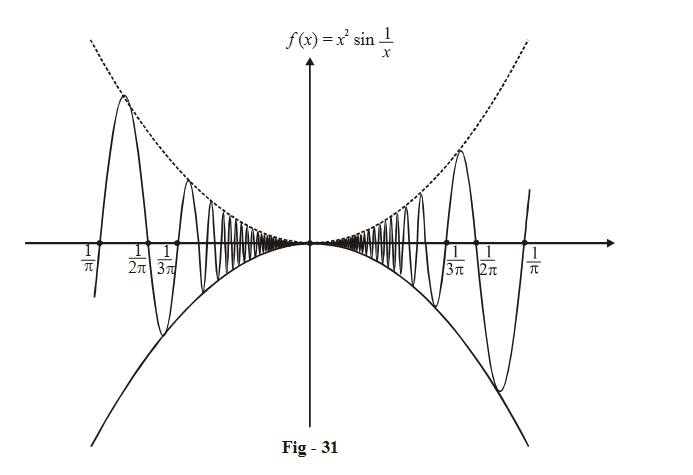Figures 30 and 31 show the approximation variation we should expect for these two functions.

Notice how  the lines y = ± x  ‘envelope’ the graph of the function in the first case and the curve $$y = \pm \,{x^2}$$  ‘envelope’ the graph of the function in the second case.

The envelopes shrink to zero vertical width at the origin in both cases. Therefore, we must have: $\underset{x\to 0}{\mathop{\lim }}\,\,\,x\sin \frac{1}{x}=0\text{ }~and\text{ }\underset{x\to 0}{\mathop{\lim }}\,\,\,{{x}^{2}}\sin \frac{1}{x}=0\text{ }$

(This is also analytically obvious; sin $$\frac{1}{x}$$  is a finite number between –1 and 1; when it gets multiplied      by x(where x → 0), the whole product gets infinitesmally small).

Now lets try to get a ‘feel’ on what will happen to the derivatives of these two functions at the origin.

For $$f(x) = x\sin \frac{1}{x},$$  the slope of the envelope is constant (±1). Thus, the sinusoidal function inside the envelope will keep on oscillating as we approach the origin, while shrinking in width due to the shrinking envelope. The slope of the curve also keeps on changing and does not approach a fixed value.

However, for $$g(x) = {x^2}\sin \frac{1}{x},$$  the slope of the envelope is itself decreasing as we approach the origin, apart from shrinking in width. This envelope will ‘compress’ or ‘hammer out’ or ‘flatten’ the sin oscillations near the origin. What should therefore happen to the derivative? It should become 0 at the origin!

Let us ‘zoom in’ on the graphs of both the functions around the origin, to see what is happening: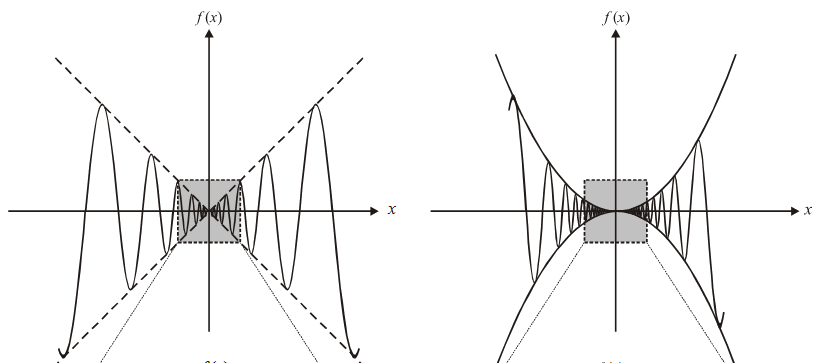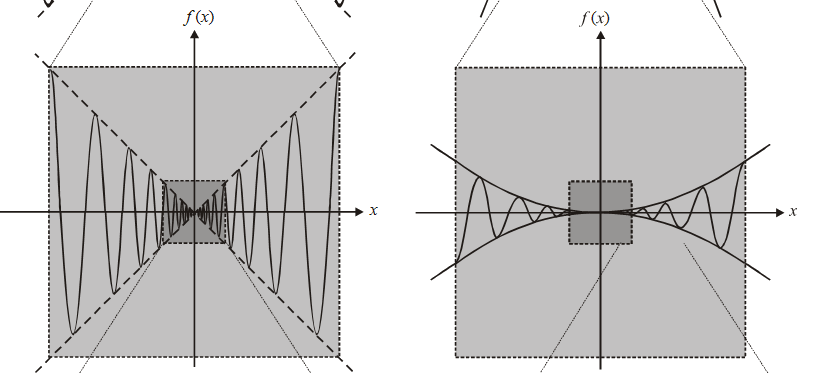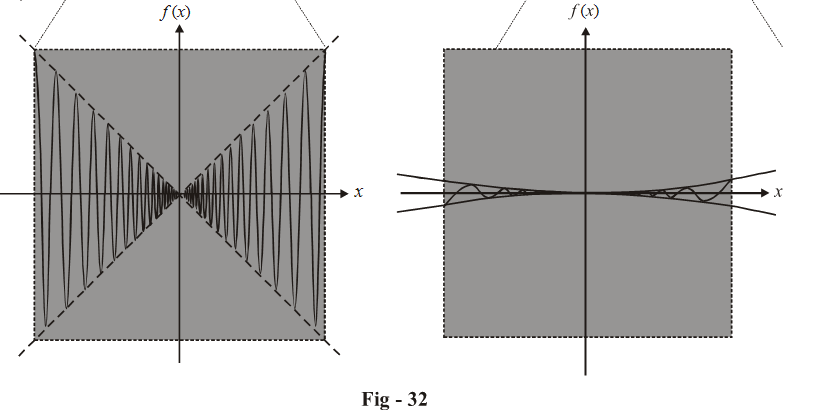These graphs are not very accurate and are only of  an approximate nature; but they do give us some feeling on the behaviour of these two functions near the origin.

The \begin{align}x\sin \frac{1}{x}\end{align} graph keeps ‘continuing’ in the same manner no matter how much we zoom in; however, in the  \begin{align}{x^2}\sin \frac{1}{x}\end{align}  graph, the decreasing slope of the envelope itself tends to flatten out the curve and make its slope tend to 0. Hence, the derivative of   \begin{align}x\sin \frac{1}{x}\end{align}  at the origin will not have any definite value, while the derivative of \begin{align}{x^2}\sin \frac{1}{x}\end{align} will be 0 at the origin .

Lets verify this analytically:

(i) $$\boxed{f\left( x \right) = x\sin \frac{1}{x}{\text{at }}x = 0}$$

$${\rm{LHD = RHD}} = \mathop {\lim }\limits_{h \to 0} \frac{{f\left( h \right) - f\left( 0 \right)}}{h}$$

\begin{align}= \mathop {\lim }\limits_{h \to 0} \frac{{h\sin \frac{1}{h}}}{h}\\= \mathop {\lim }\limits_{h \to 0} \left( {\sin \frac{1}{h}} \right)\end{align}

This limit, as we know, does not exist; hence, the derivative for f (x) does not exist at x = 0

(ii) $$\boxed{f\left( x \right) = {x^2}\sin \frac{1}{x}{\text{at }}x = 0}$$

$${\rm{LHD = RHD}} = \mathop {\lim }\limits_{h \to 0} \frac{{{h^2}\sin \frac{1}{h} - 0}}{h}$$

\begin{align} & =\underset{h\to 0}{\mathop{\lim }}\,h\sin \frac{1}{h} \\ & =\text{ }0 \\ \end{align}

This verifies our earlier assertion

Learn from the best math teachers and top your exams

• Live one on one classroom and doubt clearing
• Practice worksheets in and after class for conceptual clarity
• Personalized curriculum to keep up with school